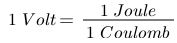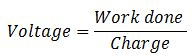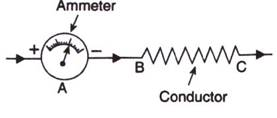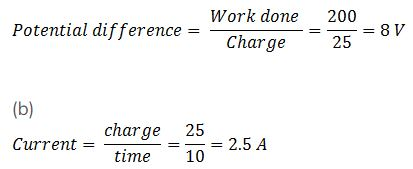## Solutions of Electricity-Lakhmir Singh Manjit Kaur VSA and LAQ Pg No. 5 Class 10 Physics1. By what other name is the unit Joule⁄Coulomb called?

→ Potential Difference is said to be 1 Volt between two points if 1 Joule of work is done in moving 1 coulomb of electric charge from one point to another.2. Which of the following statements correctly defines a volt?
(a) A volt is a joule per ampere.
(b) A volt is a joule per coulomb.

→ The second statement defines "volt" correctly.
Explanation: One Volt is the electric potential energy per unit charge, and is measured in joules per coulomb.

3A. What do the letters p.d. stand for?

→ p.d. stands for potential difference. Potential difference between two points in a circuit is the work done in moving unit charge (i.e. one coulomb) from one point to the other.

3B. Which device is used to measure p.d.?

→ Voltmeter is a device which is used to measure potential difference between two point in an electric ciruit.

4. What is meant by saying that the electric potential at a point is 1 volt?

→ Electric Potential at a point being 1 volt means that 1 joule of work is done in moving 1 unit positive charge from infinity to that point.

5. How much work is done when one coulomb charge moves against a potential difference of 1 volt?

→ 1 Joule of work is done when 1 coulomb of charge moves against a potential difference of 1 volt.
As potential difference =6. What is the SI unit of potential difference?

→ The SI unit of potential difference is volt, which is also known as Joule/Coulomb.

7. How much work is done in moving a charge of 2 C across two points having a potential difference of 12 V?

→ Given, charge = 2 C
Potential difference between two points = 12V
Work done (W) =?
We know that;
V = W/Q
Or, W = V × Q
Or, W = 12 V × 2 C = 24 J.

8. What is the unit of electric charge?

→ The SI unit of electric charge is coulomb (C).

9. Define one coulomb charge.

→ One coulomb is that amount of electric charge that repels an equal and similar charge with a force of 9 × 109 N when placed in vacuum at a distance of one metre from it.

10. Fill in the following blanks with suitable words :
(a) Potential difference is measured in …………… by using a ……….. placed in ……… across a component.
(b) Copper is a good ………. plastic is an ………..

→ (a) Potential difference is measured in volts by using a voltmeter placed in parallel across a component.
(b) Copper is a good conductor plastic is an insulator.

11. What is meant by conductors and insulators? Give two examples of conductors and two of insulators.

→ Those substance which allow electric current to pass through them easily is called conductors. Examples. Iron, copper, zinc e.t.c

Those substance which do not allow electric current to pass through them is called Insulators. Examples. Mica, Air, Paper, e.t.c.

12. Which of the following are conductors and which are insulators?
Sulphur, Silver, Copper, Cotton, Aluminium, Air, Nichrome, Graphite, Paper, Porcelain, Mercury, Mica, Bakelite, Polythene, Manganin.

→ Conductor allow electric current to pass through them, so here conductors are Silver, Copper, Aluminum, Nichrome, Graphite, Mercury, Manganin and insulator do not allow current to pass through them, so here insulators are Sulphur, Cotton, Air, Paper, Porcelain, Mica, Bakelite, Polythene.

13. What do you understand by the term “electric potential”? (or potential) at a point? What is the unit of electric potential?

→ Electronic Potentiol or Potential is defined as the work done in moving a unit positive charge from infinity to a particular point. It is denoted by V and its unit is "Volt".

14A. State the relation between potential difference, work done and charge moved.

→ Electric potential difference is known as voltage, which is equal to the work done per unit charge to move the charge between two points against static electric field.14B. Calculate the work done in moving a charge of 4 coulombs from a point at 220 volts to another point at 230 volts.

→ Here charged moved = 4 C
Potential difference = V2 – V1 =230V – 220V = 10 V
So as we know that
Work done = Potential difference × Charge moved
= 10 × 4 = 40 joule
So, the work done in moving a charge of 4 coulombs from a point at 220 volts to another point at 230 volts is 40 Joule.

15A. Name a device that helps to measure that potential difference across a conductor.

→ Voltmeter is an apparatus used to measure the potential difference or electric potential difference between two points in an electric circuit.

15B. How much energy is transferred by a 12 V power supply to each coulomb of charge which it moves around a circuit?

→ Here potential difference = 12 V
Charge moved = 1 C
Work done = Potential difference × Charge moved
= 12 × 1 = 12 joule
So 12 J energy is transferred by a 12 V power supply to each coulomb of charge which it move around a circuit.

16A. Name a device which helps to maintain potential difference across a conductor (say, a bulb).

→ A source of electricity such as cell, battery, power supply, etc. helps to maintain a potential difference across a conductor.

16B. If a potential difference of 10 V causes a current of 2 A to flow for 1 minute, how much energy is transferred?

→ As we know that
Q = I × t
Here I= 2 A and T = 1 minute = 60 sec
Thus Q = 120 C
Again as we know
V = W/Q
W = VQ
W = 10 × 120 = 1200 joule.

17A. What is an electric current? What makes an electric current flow in a wire?

→ The flow of electric charge is known as electric current. Electric current is carried by moving electrons through a conductor. It is the potential difference between the ends of the wire which make the electric charge (or current) to flow in the wire.

17B. Define the unit of electric current (or Define ampere).

→ SI unit of electric current is ampere (A). Ampere is the flow of electric charges through a surface at the rate of one coulomb per second.

18. What is an ammeter? How is it connected in a circuit? Draw a diagram to illustrate your answer.

→ An ammeter is an apparatus which is used to measure electric current in a circuit. An ammeter is always connected in series with the circuit in which the current is to be measured.19A. Write down the formula which relates electric charge, time and electric current.

→ The equation below shows the relationship between charge, current and time: charge (coulomb, C) = current (ampere, A) × time.
Q = I × t.

19B. A radio set draws a current of 0.36 A for 15 minutes. Calculate the amount of electric charge that flows through the circuit.

→ Here I = 0.36 A
Time = 15 minute = 15 × 60 = 900 sec
So Charge = 0.36 × 900 = 324 C.

20. Why should the resistance of :
(a) an ammeter be very small?
(b) a voltmeter be very large?

→ (a) An ammeter has to measure to current flowing through the circuit. Resistance offers an obstruction to the current flow. So, if the resistance of an ammeter is large, the current measured by the ammeter will be quite less as compared to the actual amount of current flowing through the circuit which is undesirable. If ammeter has zero resistance, then it will give the exact value of current. But this is not practically possible because every material has some value of internal resistance which we can't control. For this reason, ammeter must have small resistance.
(b) If current is flowing through the voltmeter, then it is not all flowing through the load, and the potential difference across the load would change when the voltmeter is added and removed. This is unfavourable. Therefore, the voltmeter must have a very high resistance so that current doesn't flow through it.

21. Draw circuit symbols for (a) fixed resistance (b) variable resistance (c) a cell (d) a battery of three cells(e) an open switch (j) a closed switch.22. What is a circuit diagram? Draw the labelled diagram of an electric circuit comprising of a cell, a resistor, an ammeter, a voltmeter and a closed switch (or closed plug key). Which of the two has a large resistance: an ammeter or a voltmeter?

→ Electric circuit is represented by drawing circuit diagrams. A diagram which indicates how different components in a circuit have been connected by using the electrical symbols for the components is called a circuit diagram.Voltmeter is an apparatus used to measure the potential difference or electric potential difference between two points in an electric circuit. A voltmeter has a large resistance.

23. If the charge on an electron is 1.6 x I0-19 coulombs, how many electrons should pass through a conductor in 1 second to constitute 1-ampere current?

→ We know that charge over 1 electron = 1.6 × 10-19 coulomb
Thus, 1.6 × 10-19 C of charge = 1 electron
Therefore, 1 C of charge.

24. The p.d. across a lamp is 12 V. How many joules of electrical energy are changed into heat and light when:
(a) a charge of 1 C passes through it?
(b) a charge of 5 C passes through it?
(c) a current of 2 A flows through it for 10 s?

→ As we know that
Work done = potential difference x charge
a) W= 12 ×1= 12 j
b) W= 12 ×5 = 60 j
c) W = 12 × 2 x 10 = 240 j.

25. In 10 s, a charge of 25 C leaves a battery, and 200 J of energy are delivered to an outside circuit as a result.
(a) What is the p.d. across the battery?
(b) What current flows from the battery?

→ (a) As we know that26A. Define electric current. What is the SI unit of electric current.

→ The flow of electric charge is known as electric current. Electric current is carried by moving electrons through a conductor. SI unit of electric current is ampere (A).

26B. One coulomb of charge flows through any cross-section of a conductor in 1 second. What is the current flowing through the conductor?

→ One coulomb is nearly equal to 6 x 1018 electrons. SI unit of electric current is ampere (A). Ampere is the flow of electric charges through a surface at the rate of one coulomb per second. This means if 1 coulomb of electric charge flows through a cross section for 1 second, it would be equal to 1 ampere.

26C. Which instrument is used to measure electric current? How should it be connected in a circuit?

→ An ammeter is an apparatus which is used to measure electric current in a circuit. It should be connected in series with the circuit.

26D. What is the conventional direction of the flow of electric current? How does it differ from the direction of flow of electrons?

→ The direction of an electric current is by convention the direction in which a positive charge would move. Thus, the current in the external circuit is directed away from the positive terminal and toward the negative terminal of the battery. Electrons would actually move through the wires in the opposite direction i.e from negative terminal to positive terminal.

26E. A flash of lightning carries 10 C of charge which flows for 0.01 s. What is the current? If the voltage is 10 MV, what is the energy?